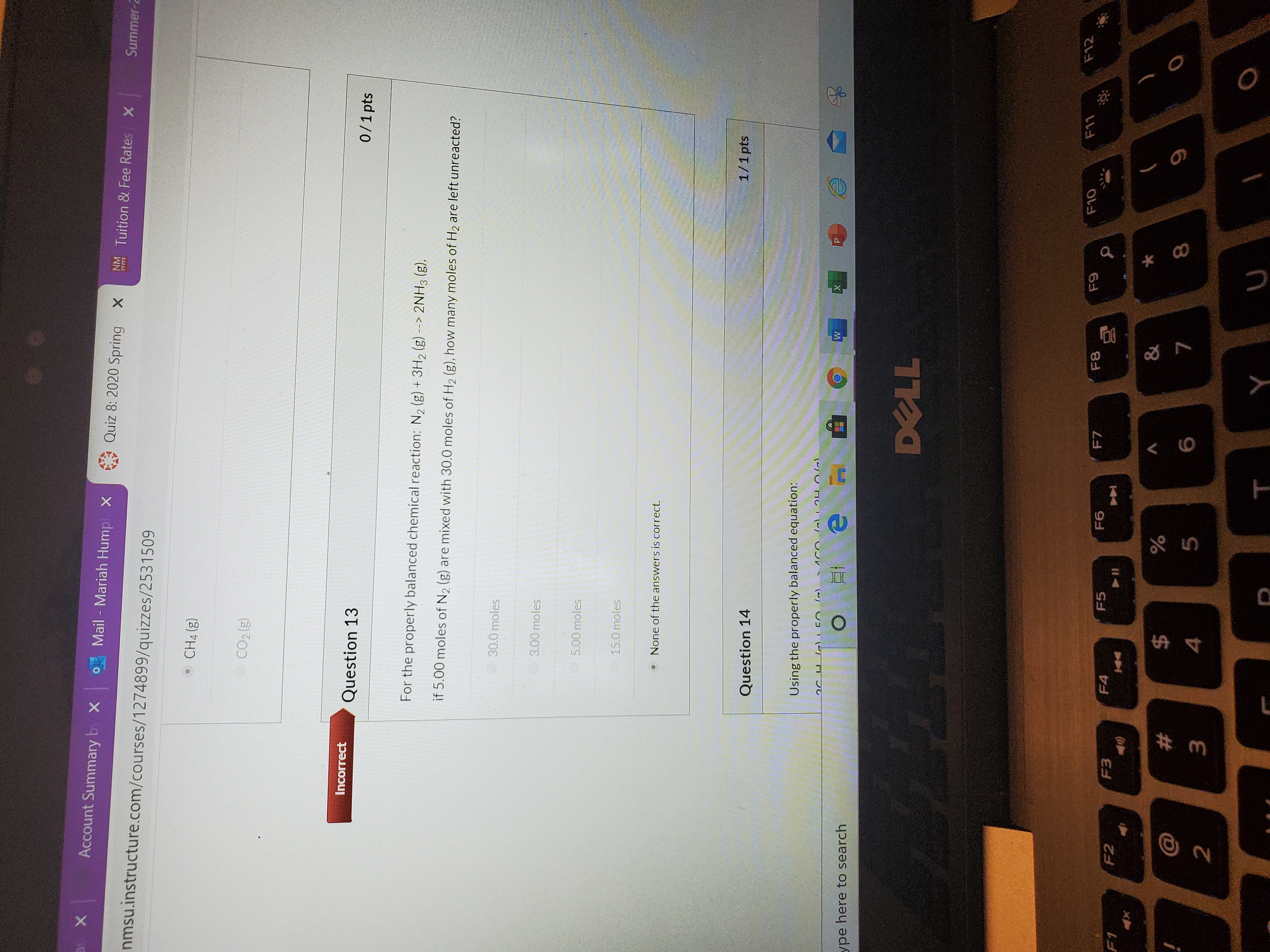# For the properly balanced chemical reaction: N2 (g) + 3 H2 (g) → 2 NH3 (g). If 5.00 mol of N2 (g) are mixed with 30.0 moles of H2 (g), how many moles of H2 are left unreacted? a. 30.0 moles b. 3.00 moles c. 5.00 moles d. 15.0 moles e. None of the answers is correct